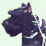# Using calculate field in a geoprocessing service

275
1
11-07-2011 04:11 PMNew Contributor
I have a model which is being published as a geoprocessing service. In one step of the model I need it to calculate a field. The field calculated is called BETA_DELTA and it is calculated as [FF_BUSINES] *%output_value% *2 with %output_value% an input that is generated by the user.

When I look at the geoprocessing results it looks like this is working as it fills in %output_value% with the user supplied number. I also made sure to have the user input as a string and then converted the data type using calculate value. However the final results show that nothing has ever been calculated.

I've had trouble with this on my very large model so I've cut it down to one where it just needs to calculate the field beta_delta. When I look at the geoprocessing results window it appears this happens, in the calculate field message it displays [FF_BUSINES] *40 *2, which makes sense because 40 is the number I input for output_value. But still nothing is calculated when I look at the results.

I'm attaching an image of my model because it might help.

So my basic question is is there a special process for using calculate field in a model that will be a geoprocessing service?
Tags (3)